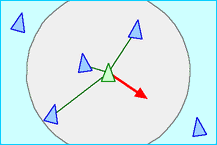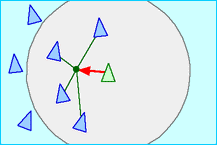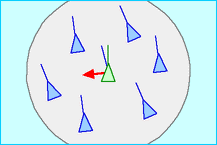# Numb3rs 515: Guilt Trip

In this episode, the jury returns a "not guilty" verdict on a case in which the plaintiff clearly showed that the defendant was guilty. Charlie analyzes how the jury was selected and how they reached the "non guilty" verdict, which leads him to believe that the jury was tempered with.

## Boid Model

Think of a flock of geese. Each goose moves independently of others, yet it tries to stay close to the rest of the flock. The movement seems synchronized, even though each bird can only see its neighbors. What rules do you think the geese follow? Boid model analyzes this movement assuming the birds have to follow only three simple rules.

In general, Boid model simulates the movement of a group of animals, such as fish schools, herds and bird flocks. The Boid model assumes three behavior rules for the animals, which are

• Avoid collision with neighbors
•• Try to be close to the neighbors: move towards the average position of local flockmates
•• Align with the neighbors: steer towards the average heading of local flockmates
•This model can be generalized by adding more rules and constraints, such as adding obstacles on the way, which the flock would have to avoid.

The Boids model is an example of an individual-based model, which is a simulation based on the global consequences of local interactions of members of a population. These more general models are being used in economics, ecology, biology and other sciences.

## Mersenne twister

Mersenne twister is a pseudorandom number generator. This very efficient algorithm which was created in 1997, resolved many issues that the earlier algorithms had. Since this algorithm is so efficient, it has been used for jury selection. The algorithm is based on Mersenne primes, which are prime numbers that can be expressed as ''power of 2 minus one'' (i.e. primes of the form 2^n-1).

Ex: 7 and 31 are Mersenne primes since 7=2^3-1 and 31=2^5-1.

"As of June 2009, only 47 Mersenne primes are known; the largest known prime number (243,112,609-1) is a Mersenne prime. In modern times, the largest known prime has almost always been a Mersenne prime. Like several previously-discovered Mersenne primes, it was discovered by a distributed computing project on the Internet, known as the Great Internet Mersenne Prime Search (GIMPS). It was the first known prime number with more than 10 million base-10 digits." http://en.wikipedia.org/wiki/Mersenne_prime
Activity 1
Mersenne numbers are all numbers of the form 2^n-1 for all positive integers n.
1. Write down fourteen smallest Mersenne numbers. (You may want to use a calculator to get some of these)
2. Which are Mersenne primes? (To do this part, you might want to recall that to verify whether a certain number is prime, you only need to check that it is not divisible by any prime smaller than or equal to its square root. Ex: To check whether 53 is a prime, you only need to make sure that it is not divisible by 2, 3, 5, or 7.)
3. Do you see any pattern?

The huge open question in this subject is:

Are there infinitely many Mersenne primes? (Remember: only 47 are known!).

Let's look at one fairly simple theorem about Mersenne primes.

Theorem:

If for a positive integer n, 2^n-1 is prime, then n has to be prime.

Proof:

Note first that if r and s are positive integers, then we have

x^[rs]-1= (x^s-1) (x^[s(r-1)] + x^[s(r-2)] + ... + x^s + 1) (Check this!).

So if n is composite (say n=rs with r and s strictly between 1 and n), then

2^n-1=2^[rs]-1= (2^s-1) (2^[s(r-1)] + 2^[s(r-2)] + ... + 2^s + 1)

is also composite (because it is divisible by 2^s-1). Q.E.D.

Thus, when looking for Mersenne primes we only need to check the expressions 2^n-1 for n prime.

Reference for pictures: http://www.red3d.com/cwr/boids/